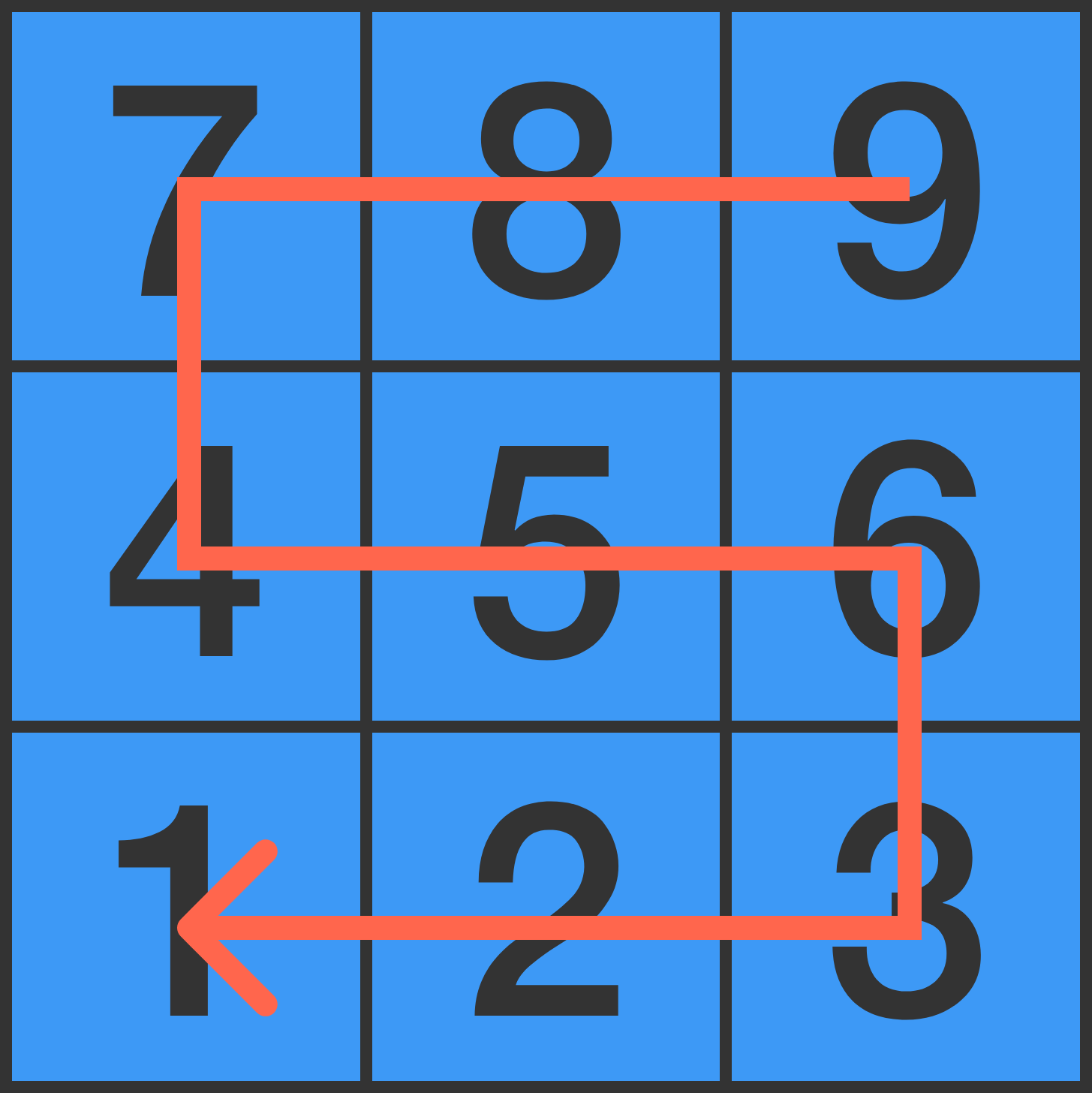On this number pad, a nine-digit number is made by walking through each digit once, starting from a random digit and only moving to adjacent digits.That number is then divided by its first digit. In this example, ${ \color{#EC7300}9}87456321 ÷ {\color{#EC7300}9} = 109717369.$ Will this method always result in an integer?

×